# Set M-values to cumulative length of line via SQL (ST_GEOMETRY)

393
2
01-31-2022 07:11 AM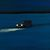by
Frequent Contributor II

ArcGIS 10.7.1; Oracle 18c; SDE.ST_GEOMETRY:

I have existing SDE.ST_GEOMETRY polylines that have M-values (aka 'measure values' for linear referencing purposes).

I want to UPDATE the M-values via Oracle SQL. In my case, the M-values should be the same as the cumulative length of the line. In other words, if we look at the image below, the M-values should be the same as the length of the line at a given vertex.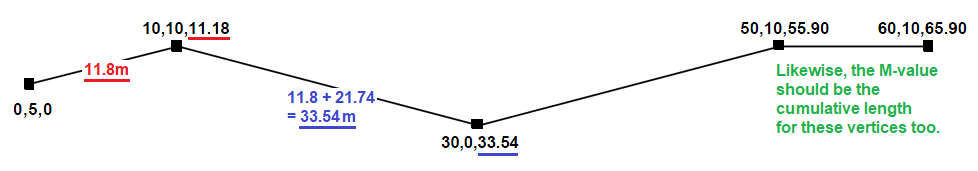Using SQL, is there a way to set the M-values to the cumulative length of the line? I'm specifically looking for an Oracle SQL solution.

Thanks.

Tags (2)
2 Replies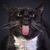Occasional Contributor III

Just to be clear in my head.... You want to convert the vertices defining your line to points and then calculate the length of the line up to that point into an M-Value field?  Are you actually using linear referencing or was that just an example or are the vertices just vertices along a linear feature?by
Frequent Contributor II

Re: "You want to convert the vertices defining your line to points and then calculate the length of the line up to that point into an M-Value field?"

Actually no, I don't want to convert the vertices to points. I want to update the M-values that are stored in the vertices of the polyline. M-values are similar to the X, Y, and Z coordinates of a given vertex...X, Y, Z, and M are stored in the SHAPE column of the feature (not in numeric fields/attributes).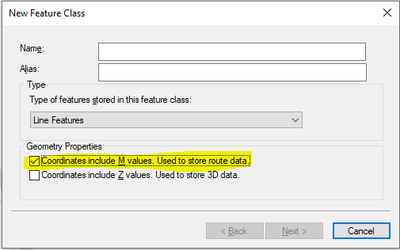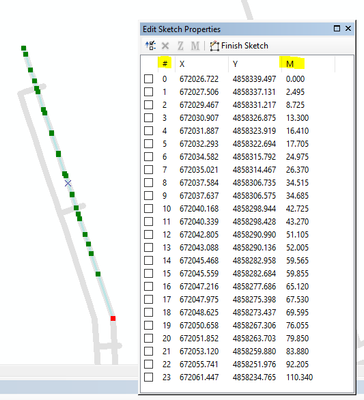"Are you actually using linear referencing or was that just an example or are the vertices just vertices along a linear feature?"

Yes, we're actually using linear referencing. We have a CONSTRUCTION_PROJECTS table (non-spatial) that has EVT_FROM and EVT_TO fields. We use that table to plot construction events along portions of lines via a linear referencing event layer (more info here).

In our case, our M-values are always exactly the same as the cumulative distance of the line. If we could, we wouldn't even use the M-values, and just use the length of the line. But I don't think that's possible. So we want to find a way to automatically set the M-values to the cumulative length of line. If I can figure out how to do it with SQL, then I'll use that SQL to create a Oracle trigger that will update the M-values if a user creates a new line or edits the shape of an existing line. That would be a lot better than relying on a user to do it manually when creating or editing a line: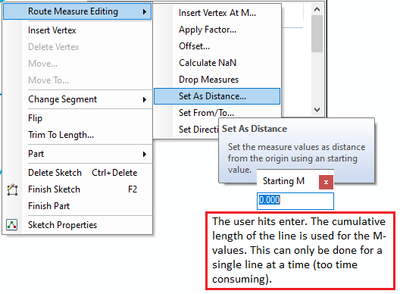Cheers.